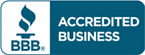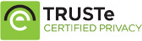# Test bank for Calculus Concepts and Contexts 4th Edition by James Stewart

\$30.00

Calculus Concepts and Contexts 4th Edition by James Stewart Test bank Questions

Full Chapters are includedCategories: ,

## Description

Test bank for Calculus Concepts and Contexts 4th Edition by James Stewart

reface.
To the Student.
Diagnostic Tests.
A Preview of Calculus.
1. FUNCTIONS AND MODELS.
Four Ways to Represent a Function. Mathematical Models: A Catalog of Essential Functions. New Functions from Old Functions. Graphing Calculators and Computers. Exponential Functions. Inverse Functions and Logarithms. Parametric Curves. Laboratory Project: Running Circles around Circles. Review. Principles of Problem Solving.
2. LIMITS AND DERIVATIVES.
The Tangent and Velocity Problems. The Limit of a Function. Calculating Limits Using the Limit Laws. Continuity. Limits Involving Infinity. Derivatives and Rates of Change. Writing Project: Early Methods for Finding Tangents. The Derivative as a Function. What Does f  Say about f ? Review. Focus on Problem Solving.
3. DIFFERENTIATION RULES.
Derivatives of Polynomials and Exponential Functions. Applied Project: Building a Better Roller Coaster. The Product and Quotient Rules. Derivatives of Trigonometric Functions. The Chain Rule. Laboratory Project: Bézier Curves. Applied Project: Where Should a Pilot Start Descent? Implicit Differentiation. Inverse Trigonometric Functions and their Derivatives. Derivatives of Logarithmic Functions. Discovery Project: Hyperbolic Functions. Rates of Change in the Natural and Social Sciences. Linear Approximations and Differentials. Laboratory Project: Taylor Polynomials. Review. Focus on Problem Solving.
4. APPLICATIONS OF DIFFERENTIATION.
Related Rates. Maximum and Minimum Values. Applied Project: The Calculus of Rainbows. Derivatives and the Shapes of Curves. Graphing with Calculus and Calculators. Indeterminate Forms and l’Hospital’s Rule. Writing Project: The Origins of l’Hospital’s Rule. Optimization Problems. Applied Project: The Shape of a Can. Newton’s Method. Antiderivatives. Review. Focus on Problem Solving.
5. INTEGRALS.
Areas and Distances. The Definite Integral. Evaluating Definite Integrals. Discovery Project: Area Functions. The Fundamental Theorem of Calculus. Writing Project: Newton, Leibniz, and the Invention of Calculus. The Substitution Rule. Integration by Parts. Additional Techniques of Integration. Integration Using Tables and Computer Algebra Systems. Discovery Project: Patterns in Integrals. Approximate Integration. Improper Integrals. Review. Focus on Problem Solving.
6. APPLICATIONS OF INTEGRATION.
More about Areas. Volumes. Discovery Project: Rotating on a Slant. Volumes by Cylindrical Shells. Arc Length. Discovery Project: Arc Length Contest. Average Value of a Function. Applied Project: Where To Sit at the Movies. Applications to Physics and Engineering. Discovery Project: Complementary Coffee Cups. Applications to Economics and Biology. Probability. Review. Focus on Problem Solving.
7. DIFFERENTIAL EQUATIONS.
Modeling with Differential Equations. Direction Fields and Euler’s Method. Separable Equations. Applied Project: How Fast Does a Tank Drain? Applied Project: Which Is Faster, Going Up or Coming Down? Exponential Growth and Decay. Applied Project: Calculus and Baseball. The Logistic Equation. Predator-Prey Systems. Review. Focus on Problem Solving.
8. INFINTE SEQUENCES AND SERIES.
Sequences. Laboratory Project: Logistic Sequences. Series. The Integral and Comparison Tests; Estimating Sums. Other Convergence Tests. Power Series. Representations of Functions as Power Series. Taylor and Maclaurin Series. Laboratory Project: An Elusive Limit. Writing Project: How Newton Discovered the Binomial Series. Applications of Taylor Polynomials. Applied Project: Radiation from the Stars. Review. Focus on Problem Solving.
9. VECTORS AND THE GEOMETRY OF SPACE.
Three-Dimensional Coordinate Systems. Vectors. The Dot Product. The Cross Product. Discovery Project: The Geometry of a Tetrahedron. Equations of Lines and Planes. Laboratory Project: Putting 3D in Perspective. Functions and Surfaces. Cylindrical and Spherical Coordinates. Laboratory Project: Families of Surfaces. Review. Focus on Problem Solving.
10. VECTOR FUNCTIONS.
Vector Functions and Space Curves. Derivatives and Integrals of Vector Functions. Arc Length and Curvature. Motion in Space: Velocity and Acceleration. Applied Project: Kepler’s Laws. Parametric Surfaces. Review. Focus on Problem Solving.
11. PARTIAL DERIVATIVES.
Functions of Several Variables. Limits and Continuity. Partial Derivatives. Tangent Planes and Linear Approximations. The Chain Rule. Directional Derivatives and the Gradient Vector. Maximum and Minimum Values. Applied Project: Designing a Dumpster. Discovery Project: Quadratic Approximations and Critical Points. Lagrange Multipliers. Applied Project: Rocket Science. Applied Project: Hydro-Turbine Optimization. Review. Focus on Problem Solving.
12. MULTIPLE INTEGRALS.
Double Integrals over Rectangles. Iterated Integrals. Double Integrals over General Regions. Double Integrals in Polar Coordinates. Applications of Double Integrals. Surface Area. Triple Integrals. Discovery Project: Volumes of Hyperspheres. Triple Integrals in Cylindrical and Spherical Coordinates. Applied Project: Roller Derby. Discovery Project: The Intersection of Three Cylinders. Change of Variables in Multiple Integrals. Review. Focus on Problem Solving.
13. VECTOR CALCULUS.
Vector Fields. Line Integrals. The Fundamental Theorem for Line Integrals. Green’s Theorem. Curl and Divergence. Surface Integrals. Stokes’ Theorem. Writing Project: Three Men and Two Theorems. The Divergence Theorem. Summary. Review. Focus on Problem Solving.
APPENDIXES.
A. Intervals, Inequalities, and Absolute Values.
B. Coordinate Geometry.
C. Trigonometry.
D. Precise Definitions of Limits.
E. A Few Proofs.
F. Sigma Notation.
G. Integration of Rational Functions by Partial Fractions.
H. Polar Coordinates.
I. Complex Numbers.
J. Answers to Odd-Numbered Exercises.

## Reviews

There are no reviews yet.